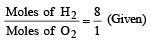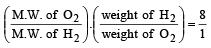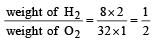Courses

# Test: States Of Matter 2 - From Past 28 Years Questions

## 30 Questions MCQ Test Chemistry Class 11 | Test: States Of Matter 2 - From Past 28 Years Questions

Description
This mock test of Test: States Of Matter 2 - From Past 28 Years Questions for NEET helps you for every NEET entrance exam. This contains 30 Multiple Choice Questions for NEET Test: States Of Matter 2 - From Past 28 Years Questions (mcq) to study with solutions a complete question bank. The solved questions answers in this Test: States Of Matter 2 - From Past 28 Years Questions quiz give you a good mix of easy questions and tough questions. NEET students definitely take this Test: States Of Matter 2 - From Past 28 Years Questions exercise for a better result in the exam. You can find other Test: States Of Matter 2 - From Past 28 Years Questions extra questions, long questions & short questions for NEET on EduRev as well by searching above.
QUESTION: 1

### If P, V, M, T and R are pressure, Volume, molar mass, temperature and gas constant respectively, then for an ideal gas, the density is given by

Solution: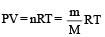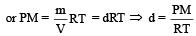QUESTION: 2

### Pressure remaining the same, the volume of a given mass of an ideal gas increases for every degree centigrade rise in temperature by definite fraction of its volume at 

Solution:

Charle’s Law - The volume of the given mass of a gas increases or decreases by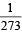of its volume at 0°C for each degree rise or fall of temperature at constant pressure.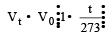at constt. Pressure

QUESTION: 3

### Correct gas equation is : 

Solution: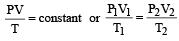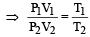QUESTION: 4

Root mean square velocity of a gas molecule is proportional to 

Solution:

According to kinetic gas equatiion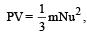u = root mean square velocity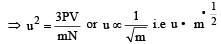QUESTION: 5

Absolute zero is defined as the temperature

Solution:

Absolute zero is the temperature at which kinetic energy of gas molecules becomes zero i.e. all molecular motion ceases.

QUESTION: 6

In vander Waal's equation of state for a non-ideal gas, the term that accounts for intermolecular forces is : 

Solution:

Vander Waal's equation for 1 mole :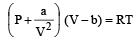Here,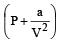represent the intermolecularforces. (V – b) is the corrected volume.

QUESTION: 7

A gas is said to behave like an ideal gas when the relation PV/T = constant. When do you expect a real gas to behave like an ideal gas ? 

Solution:

At low pressure and high temperature: At low pressure volume correction for 1 mole of a gas in negligible, i.e b = 0 thus the gas equation becomes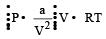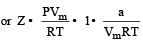At higher pressure, the pressure correction for 1 mole of gas in negligible i.e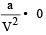or (P + 0) (V – b) = RT
or P (Vm – b) = RT
or PVm = RT + Pb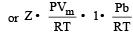QUESTION: 8

In a closed flask of 5 litres, 1.0 g of H2 is heated from 300 to 600 K. Which statement is not correct?

Solution:

Here volume is constant. Again the mass of H2 is fixed so the number of moles of the gas do not change. As temperature increases the pressure also increases. The rate of collision among the gas molecules and their energy also increases.

QUESTION: 9

At constant temperature, for a given mass of an ideal gas 

Solution:

According to Boyle’s law at constant temperature,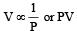= constant

QUESTION: 10

The root mean square speeds at STP for the gases H2, N2, O2 and HBr are in the order : 

Solution: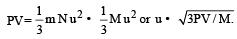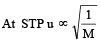i.e higher will be the molar mass lower will be the value of urms.
Molecular masses of H2, N2, O2 and HBr are 2, 28, 32 and 81. Hence the correct order is HBr < O2 < N2 < H2

QUESTION: 11

A closed flask contains water in all its three states solid, liquid and vapour at 0°C. In this situation, the average kinetic energy of water molecules will be

Solution:

Velocity and hence the K.E is  maximum in the gaseous state.

QUESTION: 12

Which is not true in case of an ideal gas ? 

Solution:

Molecules in an ideal gas move with different speeds.

QUESTION: 13

The correct value of the gas constant ‘R’ is close to :

Solution:

R = 0.082 litre atm K–1 mole–1 .

QUESTION: 14

An ideal gas can’t be liquefied because 

Solution:

In the ideal gas, the intermolecular forces of attraction are negligible and hence it cannot be liquefied.

QUESTION: 15

Select one correct statement. In the gas equation, PV = nRT 

Solution:

In the equation PV = nRT, n moles of the gas have volume V.

QUESTION: 16

At STP, 0.50 mol H2 gas and 1.0 mol He gas

Solution:

Average kinetic energy depends only on temperature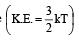QUESTION: 17

Under what conditions will a pure sample of an ideal gas not only exhibit a pressure of 1 atm but also a concentration of 1 mole litre–1 ? (R = 0.082 litre atm mol–1deg–1) 

Solution: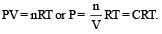Hence 1 = 1 × 0.082 × T is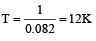QUESTION: 18

Internal energy and pressure of a gas per unit volume are related as : 

Solution: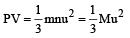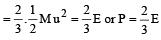per unit vol.

QUESTION: 19

The ratio among most probable velocity, mean velocity and root mean square velocity is given by


Solution:

Most probable velocity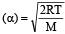Mean velocity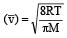Root mean square velocity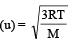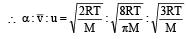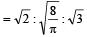QUESTION: 20

When is deviation more in the behaviour of a gas from the ideal gas equation PV = nRT ? 

Solution:

At low temperature and high pressure.

QUESTION: 21

The temperature of the gas is raised from 27°C to 927°C, the root mean square velocity is 

Solution: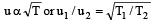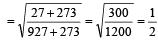u2 = 2u1

QUESTION: 22

In a pair of immiscible liquids, a common solute dissolves in both and the equilibrium is reached.Then the concentration of the solute in upper layer is

Solution:

By definition of Nernst distribution law.
When a solute is shaken with two immiscible liquids, having solubility in both, the solute distributes itself between the two liquids in such a way that the ratio of its concentrations in two liquids is constant at a given temperature, provided the molecular state of the solute remains the same in both the liquids.

QUESTION: 23

A liquid can exist only : 

Solution:

A substance exists as a liquid above its m. pt. and below its b. pt.

QUESTION: 24

500 ml of nitrogen at 27°C is cooled to –5°C at the same pressure. The new volume becomes

Solution:

Given initial volume (V1) = 500 ml ; Initial temperature (T1) = 27ºC = 300 K and final temperature (T2) = –5ºC = 268 K.
From Charle’s law :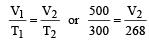Where V2 = New volume of gas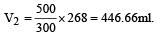QUESTION: 25

600 c.c. of a gas at a pressure of 750 mm is compressed to 500 c.c. Taking the temperature to remain constant, the increase in pressure, is

Solution:

Given initial volume (V1) = 600 c.c.;
Initial pressure (P1) = 750 mm and final volume (V2) = 500 c.c. according to Boyle’s law,

P1V1 = P2V2
or 750 × 600 = P2 × 500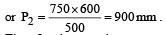Therefore increase in pressure = (900 – 750)

= 150 mm.

QUESTION: 26

Cyclopropane and oxygen at partial pressures 170 torr and 570 torr respectively are mixed in a gas cylinder. What is the ratio of the number of moles of cyclopropane to the number of moles of oxygen (nC3H6/nO2)? 

Solution:

By Ideal gas equation P1V =  n1RT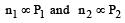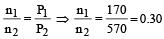QUESTION: 27

At which one of the following temperatur e -pressure conditions the deviation of a gas from ideal behaviour is expected to be minimum? 

Solution:

At low pressure and high temperature real gas nearly behave like ideal gas. Hence deviation is minimum from ideal behaviour.

QUESTION: 28

From a heated mixture of nitrogen, oxygen and carbon, two compounds (out of the many obtained) are isolated. The rates of diffusion of the two isolated compounds are almost identical.The two compounds are 

Solution:

Rate of diffusion depend upon molecular weight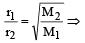r1 = r2 if M1 = M2

Hence compounds are N2O and CO2 as both have same molar  mass i.e 22

QUESTION: 29

If 500 ml of gas A at 400 torr and 666.6 ml of B at 600 torr are placed in a 3 litre flask, the pressure of the system will be 

Solution:

Applying Boyle's law P1V1 = P2V2 for both gase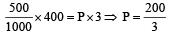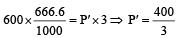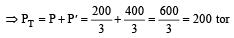QUESTION: 30

A gaseous mixture contains H2 and O2 in the molar ratio 8 : 1. The ratio of H2 : O2 by weight in this mixture would be 

Solution: